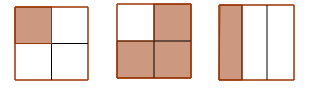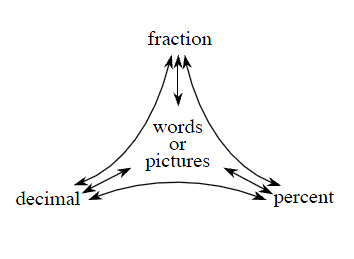### Home > MC2 > Chapter 3 > Lesson 3.2.1 > Problem3-64

3-64.

Complete a Portions Web, described in Lesson 1.1.5, for each number below.If each number value can be represented by the diagrams above, how can you rewrite them to complete the portions web?

a. $0.25$

$25 \% \text{, one fourth or } \frac{1}{4}$

b. $\frac { 3 } { 4 }$

c. $33 \frac { 1 } { 3 } \%$